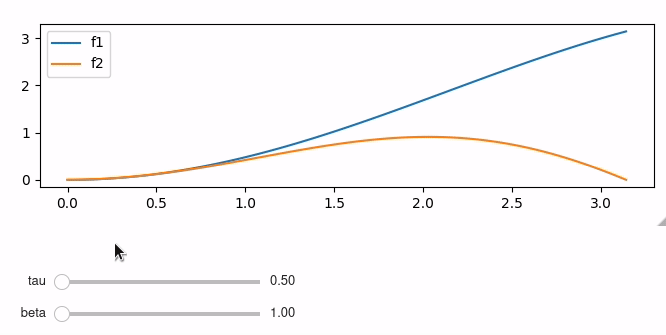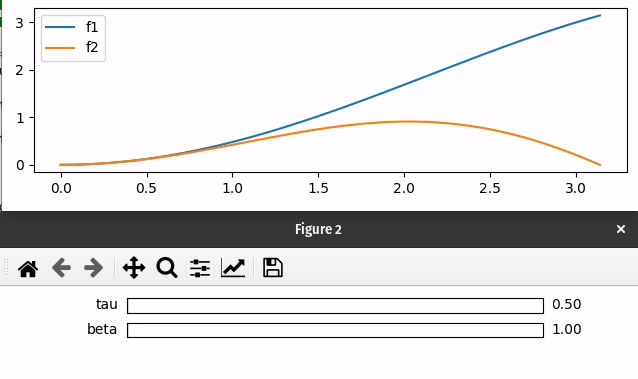# mpl_interactions: Easy interactive Matplotlib plots¶

mpl_interactions’ aims to make it as easy as possible to create responsive Matplotlib plots. In particular, you can:

• Better understand a function’s change with respect to a parameter.

• Visualize your data interactively.

To achieve this, mpl_interactions provides:

• A way to control the output of pyplot functions (e.g. `plot` and `hist`) with sliders

• A function to compare horizontal and vertical slices of heatmaps.

• A function allowing zooming using the scroll wheel.

## Installation¶

To install, simply run: `pip install mpl_interactions`

To also install version of `ipympl` and `ipywidgets` that are known to work install the optional jupyter dependencies by running `pip install mpl_interactions[jupyter]`

Further instructions for installation from JupyterLab can be found on the Installation page.

## Getting Help¶

If you have a question on how to do something with `mpl_interactions` a great place to ask it is: https://discourse.matplotlib.org/c/3rdparty/18. Feel free to mention `@ianhi` in your post there.

## Basic example¶

To control a plot with a slider:

```# if running this code in a Jupter notbeook or JupyterLab
%matplotlib ipympl
```
```import mpl_interactions.ipyplot as iplt
import matplotlib.pyplot as plt
import numpy as np

x = np.linspace(0, np.pi, 100)
tau = np.linspace(0.5, 10, 100)

def f1(x, tau, beta):
return np.sin(x * tau) * x * beta
def f2(x, tau, beta):
return np.sin(x * beta) * x * tau

fig, ax = plt.subplots()
controls = iplt.plot(x, f1, tau=tau, beta=(1, 10, 100), label="f1")
iplt.plot(x, f2, controls=controls, label="f2")
_ = plt.legend()
plt.show()
```

If you are in a Jupyter Notebook the output will look like this:and from a script or ipython the output will use Matplotlib sliders:## Matplotlib backends¶

mpl_interactions’ functions will work in any Matplotlib backend. In most backends they will use the Matplotlib Slider and Radio button widgets. However, if you are working in a Jupyter notebook the ipympl backend then ipywidgets sliders will be used as the controls. Further discussion of the behavior as a function of backend can be found on the Matplotlib Backends page.

Follow the links below for further information on installation, functions, and plot examples.